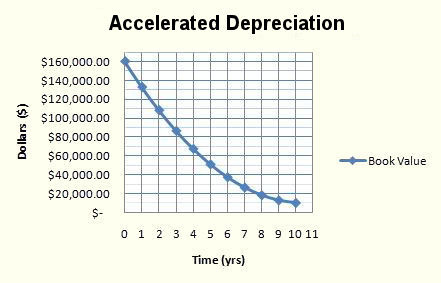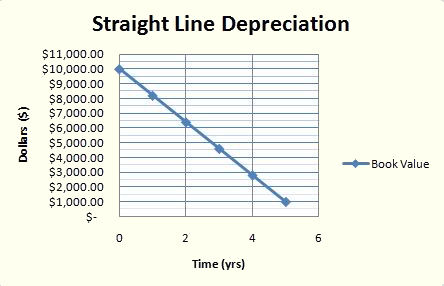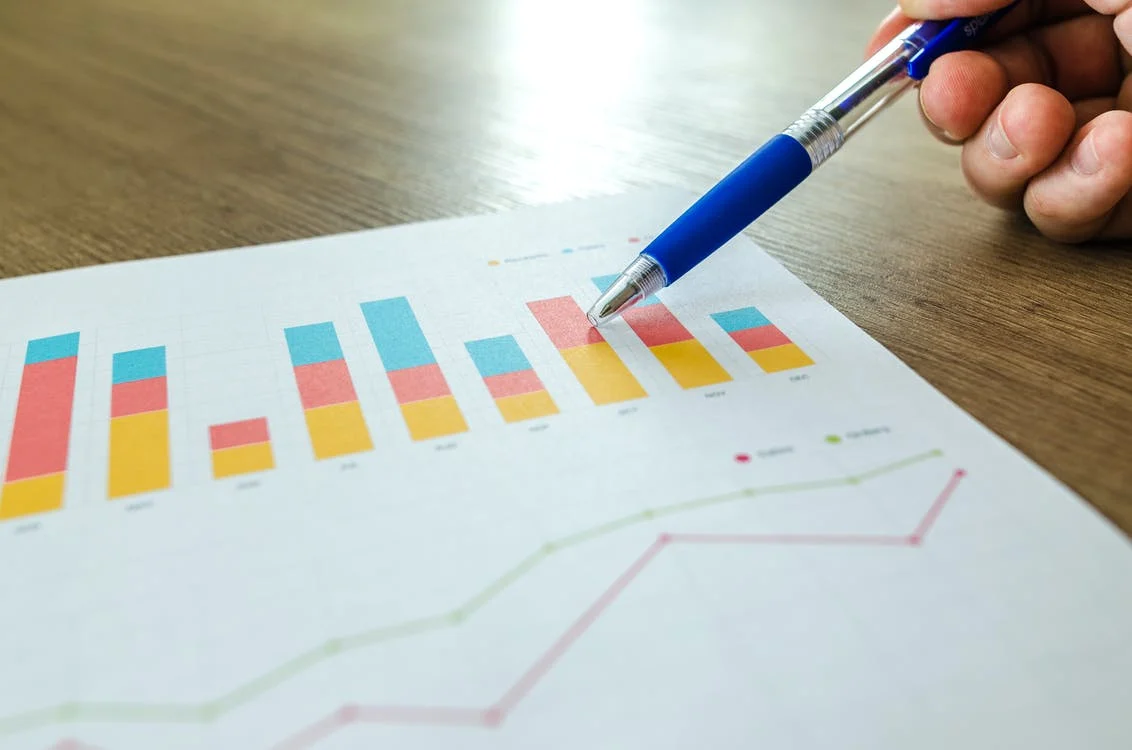# Accelerated Depreciation

What is Accelerated Depreciation?

Accelerated depreciation is a method of depreciation in which a company depreciates a fixed asset such that the amount of annual depreciation is higher during earlier years as compared to the later years of an asset's useful life.It is commonly used for two purposes; financial accounting and tax filing. In financial accounting, accelerated depreciation is used when the asset is expected to be more productive during its earlier years, thereby ensuring that the cost of production properly accounts for the additional productivity.It provides a way of deferring income taxes by delaying the taxes payable to the future for tax purposes. Since depreciation is tax-deductible, higher deductions upfront mean lower taxable profits, which means lower taxes.

It is a valuable tax incentive that encourages businesses to purchase new assets, thereby contributing to a country's growth. However, its downside is that it is often misused as part of tax reduction strategies.

## How does Accelerated Depreciation work?

Depreciation means reducing the value of a fixed asset used for business purposes, such as manufacturing products. The wear and tear associated with the usage of that asset will lower its value over time. For accounting purposes, this is an expense to the business owner.

The question, however, is how to recognize this expense? For example, would we share the expense over each period of an asset's life equally? Or would we depreciate its value based on how much it is actually used? Or maybe a better way would be to depreciate it only when it encounters factors that cause it to depreciate (like an airplane's landing gear that depreciates with each landing).

The first method, where we calculate the reduction in the value of an asset by dividing its value by its useful life in years, is called straight-line depreciation.

For instance, with straight-line depreciation, if you pay \$20,000 for a truck and expect it to have value for 10 years, the depreciation expense will be \$2,000 per year. This method of depreciation is the simplest and most commonly used method.

On the other hand, accelerated depreciation assumes that the value of assets reduces faster in the first few years of service due to high use than in the later years.Let's understand this better with the help of an example. Max, the owner of Banana Inc, purchased a machine for peeling a certain type of banana. Unfortunately, due to its design, it slowly starts to lose productivity. As a brand new asset, it can peel 10,000 bananas a day, whereas five years from now, it is expected only to be able to peel 3,000 a day.

This is a drastic reduction in productivity, and hence it would be unfair to account for depreciation at the same rate across the whole period. Therefore, these are the situations where accelerated depreciation is preferred over straight-line depreciation.

## When to use Accelerated Depreciation?

Accelerated depreciation is the preferred choice for assets whose productivity reduces over time. An example of such a situation is discussed in the previous section on Banana Inc.

However, those are not the only situations where one might be inclined to use accelerated depreciation. Remember, the second purpose for using accelerated depreciation revolved around deferring taxes.

To illustrate this better, let's look at an example. Firoz earns \$50,000 in gross income, reported on a W-2 form to the Internal Revenue Service (IRS). At a federal tax rate of 20 percent, the income tax liability of Firoz's salary is \$10,000.

On his W-4 filing, Firoz's employer withheld \$8,000 in federal taxes, and Firoz made a \$1,000 tax payment during the year. When he files his Form 1040 individual tax return, the remaining tax payment due is \$1,000. Alternatively, if Firoz's employer only withheld \$5,000 and he made no additional payments, Firoz now owes the IRS \$5,000.

## Methods of Accelerated Depreciation

There are quite a few ways to calculate accelerated depreciation. This article will cover the two most commonly used methods to account for it.

### Method 1: Double-Declining Balancing Method:In this method, the useful life is first calculated, after which it is doubled. We then take the reciprocal of the doubled amount as the annual depreciation rate. An important point to note is that the rate is applied on the written-down value at the start of the year and not the asset's cost (unlike straight-line depreciation).

The formula for calculating the depreciation rate is as follows:

Depreciation rate = 2 * (1 / useful life of the asset)

The formula for calculating the annual depreciation amount after calculating the depreciation rate is as follows.

Depreciation amount = Opening balance of net fixed asset * Depreciation rate

Let's look at an example to understand this better.

Let's assume that a farmer purchases a tractor for \$40,000, which has a useful life of 5 years.

The depreciation rate is 40% (2 * (1 / 5)). The depreciation amount for the first year would amount to \$16,000 (\$40,000 * 40%). For the second year, however, depreciation would be calculated on \$24,000 (\$40,000 - \$16,000) and not the cost which is \$40,000.

The table below illustrates the depreciation expense over the life of the tractor.

Example of Depreciation: Double-Declining Balancing Method
End of YearOpening Net Book Value(NBV)Depreciation (Opening NBV*40%)Closing Net Book Value (NBV)

1

\$40,000.00

\$16,000.00

\$24,000.00

2

\$24,000.00

\$9,600.00

\$14,400.00

3

\$14,400.00

\$5,760.00

\$8,640.00

4

\$8,640.00

\$3,456.00

\$5,184.00

5

\$5,184.00

\$2,073.60

\$3,110.40

### Method 2: Sum of the year's digit

The sum-of-the-years'-digits (SYD) is an alternative to the DDB method of accelerated depreciation.

It is best understood with the help of an example. First, we sum up all the digits of each year in the life of an asset. For an asset having a useful life of five years, each of its years would be 1, 2, 3, 4, and 5. The sum of them is 15, which we will use as the base.

The next step is to use the highest year as the numerator for the first year, the second-highest for the second, etc. In this example, the first-year depreciation would be calculated as 5/15, the second year as 4/15, the third year as 3/15, the fourth year as 2/15, and the final year as 1/15, after which the balance in the books should be zero.

Let's look into a better example is Monkey company purchasing a motorcycle worth \$300,000 with an estimated salvage value of \$5,000 for a useful life of 10 years.

The initial cost will be the same throughout the year as it goes.

Sum-of-the-years'-digits Method
YearRemaining estimated useful life at the beginning of the yearSYDApplicable percentageAnnual Depreciation

1

5

5/15

33%

\$53,636

2

4

4/15

26.67%

\$48,273

3

3

3/15

20%

\$42,909

4

2

2/15

13.33%

\$37,545

5

1

1/15

6.67%

\$32,182

## Comparison between double declining method and the sum of year's digits methods

The sum-of-years-digits (SYD) method involves writing off higher depreciation expenses in the earlier years of useful life and lower depreciation in later years. The double-declining balance (DDB) method of depreciation is similar to the SYD method in this regard.

However, the DDB method involves writing off depreciation which is much higher than the SYD method in the earlier years.

Let's understand this better with the help of an example. Let's assume a particular washing machine is worth \$100,000 with a useful life of 5 years.

The table below shows the comparison between the amount of depreciation recorded using both methods.

Comparison between double declining method and sum of year's digits methods
YearOpening Book Value(DDB)Opening Book Value(SYD)Depreciation amount of(DDB)Depreciation amount of(SYD)Closing Book Value of(DDB)Closing Book Value of(SYD)

1

\$100,000

\$100,000

\$40,000

\$33,333

\$60,000

\$66,667

2

\$60,000

\$66,667

\$24,000

\$26,667

\$36,000

\$40,000

3

\$36,000

\$40,000

\$14,400

\$20,000

\$21,600

\$20,000

4

\$21,600

\$20,000

\$8,640

\$13,333

\$12,960

\$6,667

5

\$12,960

\$6,667

\$5,184

\$6,667

\$7,776

\$0### Everything You Need To Build Your Accounting Skills

To Help you Thrive in the Most Flexible Job in the World.

Researched and authored by Savan Sabu | LinkedIn